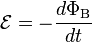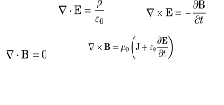# A visual representation of varying basic Electromagnetism phenomenon through GIFs

Electromagnetism is one of the four fundamental interactions in nature, the other three being the strong interaction, the weak interaction, and gravitation. This force is described by electromagnetic fields, and has innumerable physical instances including the interaction of electrically charged particles and the interaction of uncharged magnetic force fields with electrical conductors. The feature includes several animations related to electromagnetic phenomenon.

1) Lorentz ForceImage Courtesy: jnaudin.free.fr

According to Lorentz force law, there is a force F acting on a particle of electric charge q with instantaneous velocity v, due to an external electric field E and magnetic field B. The mathematical statement is given as:

F = q (E + v x B)

The electric and magnetic field vary in space and time

2) Faraday’s Law of Electromagnetic InductionImage Courtesy: onlinephys.comImage Courtesy: www.vivaxsolutions.com

The discovery of induction was made by Michael Faraday in 1831. Electromagnetic induction is the production of an electromotive force across a conductor when it is exposed to a varying magnetic field. Mathematically it is written as:Where E is the electromotive force (EMF) and ΦB is the magnetic flux.

3) Lenz’s LawImage Courtesy: www.indiegogo.com

If put simply, Lenz’s law states that if you create a changing magnetic field by moving a magnet, it will produce a mechanical force which will try to slow down the motion. The faster you move, the stronger the force gets.

4) Maxwell’s EquationImage Courtesy: en.wikipedia.orgThis set of four differential equations describes the behavior of and relationship between electricity (E) and magnetism (H). Maxwell’s equations are the foundation of our explanation of how electromagnetism works on a day to day scale.

5) Electromagnetic RadiationImage Courtesy: mason.gmu.edu

It is a basic phenomenon of electromagnetism where the radiations behave as waves as well as particles that carry radiant energy through space. EMR is emitted and absorbed by charged particles. The wave has both electric and magnetic field components that oscillate perpendicular to each other.

6) Poynting VectorImage Courtesy: en.wikipedia.org

In physics, the Poynting vector represents the directional energy flux density (the rate of energy transfer per unit area, in units of watts per square metre (W/m2)) of an electromagnetic field. The animation shows dipole radiation of a dipole vertically in the page showing electric field strength (colour) and Poynting vector (arrows) in the plane of the page.

7) Eddy CurrentsImage Courtesy: www.integratedsoft.com

Eddy currents are electric currents induced within conductors by a changing magnetic field in the conductor. These circulating eddies of current have inductance and thus induce magnetic fields. These fields can cause repulsion, attraction, propulsion, drag, and heating effects. The stronger the applied magnetic field, the greater the electrical conductivity of the conductor, and the faster the field changes, the greater the currents that are developed and the greater the fields produced.

8) Meissner EffectImage Courtesy: justgif.com

The Meissner effect is an expulsion of a magnetic field from a superconductor during its transition to the superconducting state. Superconductors in the Meissner state exhibit perfect diamagnetism, or superdiamagnetism, meaning that the total magnetic field is very close to zero deep inside them. The animation shows a magnet levitating above a superconductor cooled by liquid nitrogen.

39582   09/07/2014